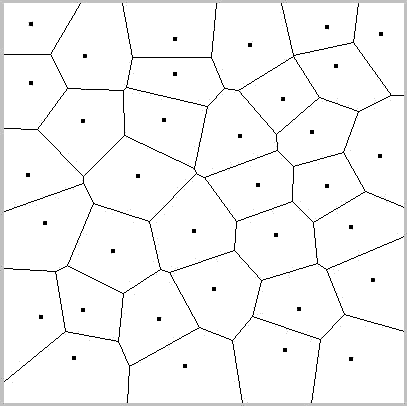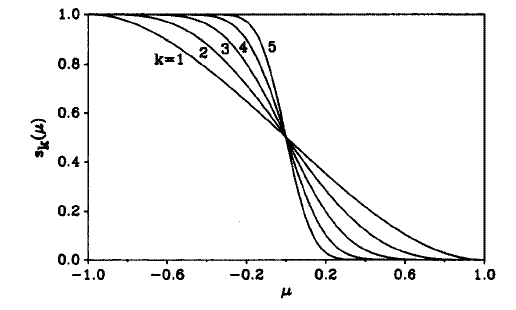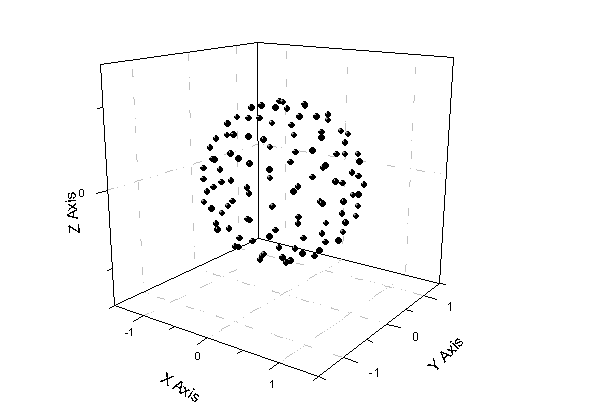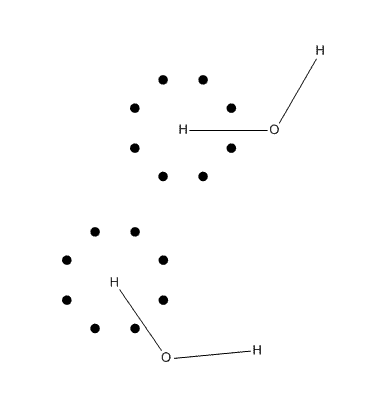# 密度泛函计算中的格点积分方法

First release: 2010-SEP-3  Last Update: 2011-Aug-23

## 2. 积分的转化W_i(r)=1  当r处于i原子空间内
W_i(r)=0  当r处于i原子空间外## 3. 单中心球极坐标的积分

### 3.1 简介

∫[0,2π]∫[0,π]∫[0,∞) f(r)*r^2*sinθdθdφdr  其中R是径向坐标

x=R*sinθ*cosφ
y=R*sinθ*sinφ
z=R*cosθ

### 3.2 角度部分积分

program ltwd
integer,parameter :: num=110
real*8,dimension(num) :: x,y,z,w
call LD0110(X,Y,Z,W,N)  //传入容量与节点数相同的一维数组接收结果。最后一个参数没什么用，随便传进一个整数即可，返回值就是生成的节点数。
do i=1,num
write(1,"(4f15.10)") x(i),y(i),z(i),w(i)
end do
end### 3.3 径向部分积分

n阶（即n个根）第二类Chebyshev多项式的每个根（即积分节点）x_i=cos(i*π/(n+1))，权重系数w_i=π/(n+1)*sin(i*π/(n+1))^2。

∑[i]2*π/(n+1)*P^3*(1+x_i)^2.5/(1-x_i)^3.5*q(R_i)

∫q(R)*R^2 dR=∑[i]w_i*q(R_i)，其中
w_i=2*π/(n+1)*P^3*(1+x_i)^2.5/(1-x_i)^3.5
R_i=(1+x_i)/(1-x_i)*P
x_i=cos(i*π/(n+1))

## 4. 积分格点的调整

s(μ)函数在自变量等于0，即两原子中垂面处为0.5，这个中垂面位置也应当根据原子共价半径做适当调整。为此，Becke将s(μ(i,j))的自变量替换为ν(i,j)，它与μ(i,j)的关系为：ν(i,j)=μ(i,j)+a(i,j)*(1-μ(i,j)^2)。目的就是找一个a(i,j)，当r_i/r_j=R_i:R_j=χ(i,j)时ν(i,j)恰为0，其中R_i和R_j为i,j原子共价半径。为此，先令ν(i,j)=0，则a(i,j)=μ(i,j)/(μ(i,j)^2-1)，在r_i/r_j=R_i:R_j时，μ(i,j)=(r_i-r_j)/R(i,j)=(r_i-r_j)/(r_i+r_j)=(χ(i,j)-1)/(χ(i,j)+1)，因此a(i,j)就确定了。推导中假设格点在i,j轴线上，所以R(i,j)=r_i+r_j。当a(i,j)小于-1/2和大于1/2时直接分别设为-1/2和1/2。笔者发现，若将所有原子设成具有相同数目的径向和角度格点，则做上述调整只会令积分精度下降，只有如下对不同原子用不同格点数目时上述调整才会提高积分精度。Becke用的是Bragg-Slater共价半径，但其中氢的半径为0.25埃，用于调整格点时显得有些过小，为得到更好结果，Becke将之改为0.35埃。

## 5. 积分代码

subroutine intfunc
use function !引用外部变量与函数
use util
implicit real*8 (a-h,o-z)
real*8,allocatable :: potx(:),poty(:),potz(:),potw(:) !接收Lebedev格点位置和权重的数组
type(content),allocatable :: gridatm(:)  !content类型在别处定义的，包含x,y,z,value四个元素，都是real*8。gridatm储存格点位置和权重
real*8 smat(ncenter,ncenter),Pvec(ncenter) !ncenter是总原子数。smat储存s(ν(i,j))，Pvec储存归一化前的w_i(r)
integer :: bondmat(ncenter,ncenter) !成键矩阵记录两个原子间是否成键，成键为1，不成键为0

maxsph=434 !假设角度部分最多有434个格点

!生成成键矩阵，原子成键数超过1就说明是内部原子，要用更多格点。
bondmat=0
do i=1,ncenter
do j=i+1,ncenter
! distmat是已算好的距离矩阵。covr第n个元素是周期表中原子序数为n的共价半径。这里用的共价半径来自Dalton Trans. 2008,2832。a(i)%index是第i个原子的原子序数。
if ( distmat(i,j) < 1.15D0*(covr(a(i)%index)+covr(a(j)%index)) ) bondmat(i,j)=1
end do
end do
bondmat=bondmat+transpose(bondmat) !成键矩阵是对称的

rintval=0 !这是将被累加的变量，由于储存最终的积分值
covr(1)=0.35D0/b2a !对氢原子用0.35埃的共价半径。由于covr记录的是以波尔为单位，用b2a=0.529177249转换单位。
call cpu_time(time_begin) !用于计算总耗时，便于研究积分格点设置与计算精度的关系。

do iatm=1,ncenter !循环每个原子
write(*,"(' Processing center',i6,'(',a2')')") iatm,a(iatm)%name !a(iatm)%name是被积分的原子的元素名
if (a(iatm)%index<=2) then
else if (a(iatm)%index<=10) then
else
end if
if (sum(bondmat(iatm,:))==1) then !判断此原子是在内侧还是边缘
sphpot=230 !设定角度部分格点数。sphpot是外部定义的整数变量。
else
sphpot=434 !内侧的原子更多的径向和角度格点
end if
if (sphpot==230) call LD0230(potx,poty,potz,potw,isphnum) !生成以0,0,0为原点，在半径为1的球面上分布的Lebedev格点坐标。
if (sphpot==434) call LD0434(potx,poty,potz,potw,isphnum)

parm=covr(a(iatm)%index)/2 !parm指的是前文(1+x_i)/(1-x_i)*P中的P
if (a(iatm)%index==1) parm=covr(a(iatm)%index) !对氢原子半径不除以2
end do

!当前积分节点是以0,0,0为中心，下面将中心转移到被积原子上
gridatm%x=gridatm%x+a(iatm)%x
gridatm%y=gridatm%y+a(iatm)%y
gridatm%z=gridatm%z+a(iatm)%z
rnowx=gridatm(i)%x !为了写的时候方便，改用rnowx,rnowy,rnowz表示
rnowy=gridatm(i)%y
rnowz=gridatm(i)%z
rfuncval=fdens(rnowx,rnowy,rnowz) !fdens是外部函数，计算此点的被积函数值，这里就是指ρ(r)。

!计算这个格点的空间划分权重W_i
smat=1.0D0
do ii=1,ncenter !为计算所有的s(ν(i,j))，循环每一对儿原子
ri=dsqrt( (rnowx-a(ii)%x)**2+(rnowy-a(ii)%y)**2+(rnowz-a(ii)%z)**2 ) !计算r_i
do jj=1,ncenter
if (ii==jj) cycle !s矩阵对角元没用，不重新赋值，值仍为1。
rj=dsqrt( (rnowx-a(jj)%x)**2+(rnowy-a(jj)%y)**2+(rnowz-a(jj)%z)**2 ) !计算r_j
rmiu=(ri-rj)/distmat(ii,jj) !计算μ(i,j)

!根据原子半径调整原子空间划分，也就是将μ(i,j)转化为ν(i,j)
chi=covr(a(ii)%index)/covr(a(jj)%index) !计算χ(i,j)
uij=(chi-1)/(chi+1)
aij=uij/(uij**2-1)
if (aij>0.5D0) aij=0.5D0
if (aij<-0.5D0) aij=-0.5D0
rmiu=rmiu+aij*(1-rmiu**2) !此时的rmiu就是ν(i,j)

tmps=1.5D0*(rmiu)-0.5D0*(rmiu)**3 !做三次迭代
tmps=1.5D0*(tmps)-0.5D0*(tmps)**3
tmps=1.5D0*(tmps)-0.5D0*(tmps)**3
smat(ii,jj)=0.5D0*(1-tmps) !得到s(ν(i,j))=0.5(1-p(p(p(ν(i,j)))))
end do
end do
Pvec=1.0D0
do ii=1,ncenter !计算W_i(r)=∏[i/=j]s(μ_i,j)。注意因为现在smat对角元为1，乘它等于没乘
Pvec=Pvec*smat(:,ii)
end do
weight=Pvec(iatm)/sum(Pvec) !做归一化，得到最终的W_i(r)
rintval=rintval+rfuncval*weight*gridatm(i)%value !原子空间划分权重weight和单中心积分权重gridatm(i)%value之积就是此点的总权重。总权重乘上此点被积函数值，对所有中心的所有节点累加，最终得到的就是积分结果。
end do
end do

!显示用时和最终结果
call cpu_time(time_end)
write(*,"(' Totally evaluated',i10,' points',' took',f8.2,' seconds')")  icount,time_end-time_begin
write(*,*)
write(*,"(' Final result:',f20.10)") rintval
end subroutine

## 6. 格点积分方法的一些问题## 附录：高斯积分

Legendre: 1  [-1,1]
Jacobi: (1-x)^a*(1+x)^b  (-1,1) 其中a,b>-1
Chebyshev first kind: 1/(1-x^2)^0.5  (-1,1)
Chebyshev second kind: (1-x^2)^0.5  [-1,1]
Laguerre: exp(-x)  [0,∞)
Hermite: exp(-x^2)  (-∞,∞)